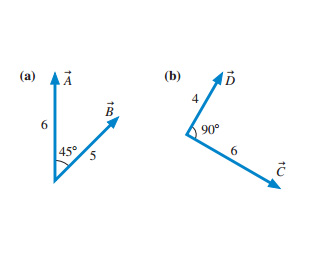# Problem: 1. Evaluate the magnitude of the cross product AxB.2. Choose the correct direction o the cross product AxB.A. Into the pageB. It is zero vecorC. It is opposite to the direction of BD. Out of the pageE. It is opposite to the direction of A3. Evaluate the magnitude of the cross product CxD.4. Choose the correct direction of the cross product CxD.A. Into the pageB. It is opposite to the direction of DC. It is zero vecorD. It is opposite to the direction of CE. Out of the page

###### FREE Expert Solution

Cross product:

$\overline{){\mathbf{A}}{\mathbf{×}}{\mathbf{B}}{\mathbf{=}}{\mathbf{A}}{\mathbf{B}}{\mathbf{s}}{\mathbf{i}}{\mathbf{n}}{\mathbf{\theta }}}$

1.

A × B = (6)(5)sin(45°) = 21.21

The magnitude of the cross product AxB is 21.21.

94% (155 ratings)###### Problem Details1. Evaluate the magnitude of the cross product AxB.

2. Choose the correct direction o the cross product AxB.

A. Into the page
B. It is zero vecor
C. It is opposite to the direction of B
D. Out of the page
E. It is opposite to the direction of A

3. Evaluate the magnitude of the cross product CxD.

4. Choose the correct direction of the cross product CxD.

A. Into the page
B. It is opposite to the direction of D
C. It is zero vecor
D. It is opposite to the direction of C
E. Out of the page